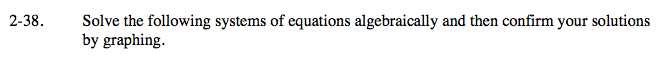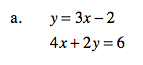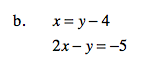Home > A2C > Chapter 2 > Lesson 2.1.3 > Problem2-38

2-38.
1. Solve the following systems of equations algebraically and then confirm your solutions by graphing. Homework Help ✎

1. y = 3x − 2
4x + 2y = 6

2. x = y − 4
2x − y = −5(1, 1)See part (a).